• 小白量化学习-自创指标设计 一、准备工作 1、首先把“HP_formula.py”文件复制到自己的工程目录中。 2、在新文件开始增加下面4条语句。 import numpy as np import pandas as pd from HP_formula import * import ...
• 自己做量化交易软件(36)小白量化实战7–小白量化回测面板设计 在股市中，没有很高文化知识的散户，也可以成为股市赢家。例如，有一位看自行车的大妈，每到一段时间存车的人很少时她就去买股票，看到一段时间存车的人...
自己做量化交易软件(36)小白量化实战9–小白量化回测面板设计 在股市中，没有很高文化知识的散户，也可以成为股市赢家。例如，有一位看自行车的大妈，每到一段时间存车的人很少时她就去买股票，看到一段时间存车的人多的时候就她去卖股票，每年都有所获。 因此散户有好的经验和技术分析的前提下，散户投资者也可以利用好的操作方法，可以在股市中经常保持获利。这个好的操作方法，例如数自行车数量，可以当作会用“股票技术指标”，技术指标是谁写的？当然是能看我博客的各位读者了。 作者出版的《看盘秘籍》不仅教炒股知识、炒股方法、炒股技术、炒股理念，还从宏观和微观介绍了股票价格波动的原理，并以此开发出荷蒲指标、独狼指标、貔貅指标系列。最后教读者如何编写大智慧和通达信软件指标公式，以及用c++来开发和加密指标的方法。
随着计算机科学的发展，投资分析由以前的简单指标分析，发展为量化投资分析技术。现在是人工智能的量化时代，没有很高文化知识的小白散户，能用小白量化软件吗？购买作者出版的<零基础搭建量化投资系统――以Python为工具>，并好评，除了得到随书提供的小白量化第一代源代码外，还赠送小白量化第二代源代码。
小白量化第二代能够编译为exe文件，做成仿通达信软件Python版，提供给没有安装过Python环境的电脑小白使用。 这篇文章给大家介绍如何定制小白量化二代的量化工具，这个工具可以称为小白量化软件的插件，增强小白量化平台的功能，更加简化用户量化分析回测。 假如小白量化目录的：d:\xb2d 小白量化中[增强插件]在view目录中 d:\xb2d\view 小白量化中[用户程序]在user目录中 d:\xb2d\user 其中文件都以.py文件存在，这些文件会在重新启动后，增加到导航目录树中。上图是小白量化二代的启动画面。这些关键控制变量与书中第一代小白量化一样。通过这些全局控制变量来控制或改变小白量化框架的外观，功能和形态。 下面我们看看[三画面.py]的插件代码。
# -*- coding: utf-8 -*-
# 显示3个K线图的模板
import time
import tkinter as tk
import HP_global as g
import HP_data as hp
from HP_view import * #菜单栏对应的各个子页面
import HP_tdx as htdx

#系统设定了g.tab1--g.tab9,系统只是用了g.tab1--g.tab6
#控件结构 g.G_root -〉 g.tabControl  -〉g.tab1
#删除tab,用forget()
#当然用户可以设置更多的tab窗口。必须使用全局变量g.变量名
#重复建立新tab窗会出错，所以我们先检测是否None,不是就先做删除旧tab窗口。

if g.tab8!=None:
g.tabControl.forget(g.tab8)
g.tab8=None

#用户自建新画面
g.tab8 = tk.Frame(g.tabControl)
g.tabControl.select(g.tab8)

#把window划分3个子容器,在不同子容器中显示不同股票K线图
xxx=view3(g.tab8)

##读取股票数据

g.gtype=g.book_s.get()
stockn=g.stock_i.get()
stockn=stockn.strip()
stockn=stockn.zfill(6)
g.stock_i.set(stockn)
g.stock=stockn
ds=g.date_s.get()
de=g.date_e.get()
g.sday=ds.strip()
g.eday=de.strip()
df2=htdx.get_k_data(g.stock,ktype='D',start=g.sday,\
end=g.eday,index=False,autype='qfq')

ds=g.mds
de2=time.strftime('%Y-%m-%d',time.localtime(time.time()))
de=time.strftime('%Y-%m-%d',time.localtime(time.time()))+' 15:01:00'
g.mde=de

stockn=g.stock_i.get()
stockn=stockn.strip()
stockn=stockn.zfill(6)
g.stock_i.set(stockn)
g.stock=stockn
st=g.stock
st2=st

if int(time.strftime('%H%M',time.localtime(time.time())))<=930:
ds=time.strftime('%Y-%m-%d',time.localtime(time.time()-24*60*60))+' 09:30:00'
de=time.strftime('%Y-%m-%d',time.localtime(time.time()-24*60*60))+' 15:00:00'
else:
ds=time.strftime('%Y-%m-%d',time.localtime(time.time()))+' 09:30:00'
de=time.strftime('%Y-%m-%d',time.localtime(time.time()))+' 15:00:00'

df2b=htdx.get_security_bars(7,code=st)
a=[x[0:10] for x in df2b.date]
df2b['date2']=a
df2b=df2b[df2b.date2==de2]
df2b=df2b.reset_index(level=None, drop=True ,col_level=0, col_fill='')

axview3x_m(xxx.v,df2b,g.stock+' '+g.stock_names[g.stock]+' 分时图',6)

df3=htdx.get_security_bars(nCategory=5,nMarket = 0,code=g.stock,\
nStart=0, nCount=240)
df3.date=[x[0:10] for x in df3.date.astype(str)]
#6均线2指标图,现实KDJ指标线
axview2x(xxx.v,df3,g.stock+' '+g.stock_names[g.stock]+' 周线图',6)
# 3指标图
axview4x(xxx.v,df2,g.stock+' '+g.stock_names[g.stock]+' 日线图',f1='VOL',f2='HPYYX',f3=g.gtype)

xxx.pack(fill=tk.BOTH, expand=1)


程序运行结果如下图：我们再看看[F10窗口.py]基本面信息的插件程序如下：
# -*- coding: utf-8 -*-
import time
import pandas as pd
import numpy as np
import tkinter as tk  #装载tkinter模块,用于Python3
from tkinter import ttk  #装载tkinter.ttk模块,用于Python3
import  HP_tk  as  htk   #导入htk
from tkinter import scrolledtext  #装载scrolledtext模块
from PIL import Image, ImageTk, ImageDraw, ImageFont
import sys
sys.path.append("..")
import HP_tk  as  htk   #导入ht
import HP_tdx as htdx
import HP_global as g

if g.tab7!=None:
g.tabControl.forget(g.tab7)
g.tab7=None

#用户自建新画面
g.tab7 = tk.Frame(g.tabControl)
mk=htdx.get_market(g.stock)

class UserF10(tk.Frame): # 继承Frame类
def __init__(self, master=None,stock=''):
tk.Frame.__init__(self, master)
self.stock=stock
if self.stock=='':
self.stock=g.stock
self.root = master #定义内部变量root
self.button2=None
self.r=1
self.c=8
self.b=[]
self.F10text=scrolledtext.ScrolledText(self,undo=True,bg='#FFF8DC')
self.init()
self.pack(fill=tk.BOTH, expand=1,)

def bt(self,n=0):
import HP_tdx as htdx
mk=htdx.get_market(self.stock)
df=htdx.get_company_info_category(mk,self.stock)
self.F10text.delete(2.0,tk.END)
self.F10text.insert(tk.INSERT,'\n\n')
t=htdx.get_F10(self.stock,df.name[n])
self.F10text.insert(tk.INSERT,t)

def bt2(self):
stockn=g.stock_i.get()
stockn=stockn.strip()
stockn=stockn.zfill(6)
self.stock=stockn
g.stock=stockn
self.init()
g.status.text(2,'查看'+self.stock+'  F10信息！')
self.bt(n=0)

def init(self):
import HP_tdx as htdx
mk=htdx.get_market(self.stock)
df=htdx.get_company_info_category(mk,self.stock)
fr=tk.Frame(self.F10text)
k=0
for i in range(self.r):
for j in range(self.c):
def kk(self=self, k=k):
self.bt(k)

self.button = tk.Button(fr,text=df.name[k],command=kk,cursor='hand2')
self.b.append(self.button)
k+=1
self.button2 = tk.Button(fr,text='切换股票',command=self.bt2,cursor='hand2')
fr.pack(side=tk.TOP)
self.F10text.window_create(tk.INSERT,window=fr)
self.F10text.insert(tk.INSERT,'\n\n')
t=htdx.get_F10(self.stock,df.name)
self.F10text.insert(tk.INSERT,t)

g.UserF10View=UserF10(g.tab7)
g.UserF10View.pack(fill=tk.BOTH, expand=1)
g.tabControl.select(g.tab7)
g.tabs=7

程序运行结果如下：无论是输入什么股票代码，敲回车后，日线图，分时图，三画图，F10基本信息，股票代码都会自动切换。 通过阅读我的文章，你是不是有更丰富的想法呢？
一位读者找到我，他的目前只会写通达信指标，一时半会学不会Python，希望花钱订制一个量化回测插件，于是我的其中一位读者为他订制了这个[小白回测]插件。使用者只需要写相应的通达信指标公式，就能实现自动回测。
#双均线策略
#买信号B
B=CROSS(MA(C,5),MA(C,20))

#卖信号S
S=CROSS(MA(C,20),MA(C,5))你觉得定制这样的插件能赚多少钱呢？一本书<零基础搭建量化投资系统――以Python为工具>全价才99元。 我相信，我们的读者看了我的帖子，设计类似的插件非常容易。
请持续关注我的博客，我的进步，就是你的进步！
展开全文python Tkinter
• 自己做量化交易软件(27)小白量化实战之1–改造兵器及显示 证券市场的多空双方，如同“武林”，里面有很多“大师”、“高手”相互博弈。当悟性和功力相当时，想要取胜就要依赖削铁如泥的“超级兵器”，例如轩辕剑、...
自己做量化交易软件(27)小白量化实战之1–改造兵器及显示
证券市场的多空双方，如同“武林”，里面有很多“大师”、“高手”相互博弈。当悟性和功力相当时，想要取胜就要依赖削铁如泥的“超级兵器”，例如轩辕剑、屠龙刀等等。有了这个“超级兵器”，才能几何倍数的发挥自己的能力，才能“笑傲武林”。
很多投资者经过在股市中的磨练，总会总结出自己的一些分析技术，甚至会创造出新的分析技术，但是自己用这些技术去选股，非常不方便，比如每只股票分析3分钟，1000只股票看完，需要50个小时，等发现了好股票，时机已经错过了。如果能利用电脑选股，才能及时把握机会。 飞狐交易师、大智慧LEVEL-2、通达信、同花顺等专业投资分析平台，提供整套分析方法设计、测试、评价、优化平台，用户可以依据炒股经验或证券分析领域的各种新思想、新方法来自己动手设计各种各样的公式系统，打造驰骋股市的秘密武器。
但是这些股票分析工具,没有资金管理,持仓管理,一揽子股票回测分析,以及套利分析回测工具,以及最终自动下单.因此Python支持深度学习,爬虫等,是量化分析首选语言,小白量化就是Python平台的量化模块。 作者在2008年出版了一本书《看盘秘籍》（https://item.jd.com/10469068.html），这本书主要介绍股票软件指标设计，以及用C++开发指标公式，凡是购买这本书的读者，除了了解作者的投资分析理念，还会得到C++开发指标公式的资料及演示程序，能够得到一套用c++开发貔貅指标（https://mp.weixin.qq.com/s/c9J-d30haP5nonU3bjiwSg），这套指标支持分析家，大智慧，飞狐交易师等软件。 本篇开始的系列文章，教大家由创造自编公式开始，到实现股票、期货、外汇、虚拟币的回测和自动交易代码。读者不难根据自己投资经验和投资理念，设计出自编指标，打造出自己的“屠龙剑”。
我们以通达信软件《广发证券金融终端》为例，来介绍打造自创兵器的过程，以及用小白量化平台模块实现回测和全自动交易。 股票软件中的指标有上千种，如果能以此赚钱，很多投资者都不是赚钱了，事实上几十年来，投资者总是一赚二平七亏损。Ta-lib集成了常用股票指标函数，因此依赖于Ta-lib做量化交易工具，也很难赚钱。 小白量化能仿通达信、大智慧、飞狐交易师等股票软件指标公式，可以实现用户自编指标的分析和回测，以及实现自动交易。购买《零基础搭建量化投资系统――以Python为工具》（https://item.jd.com/61567375505.html）一书，就能得到小白量化第二代全部源代码。 看过作者的《看盘秘籍》一书的朋友都清楚，证券投资分析宏观方面包含基本面，趋势，强弱，能量，大众心态，经济政治环境等等，在微观方面包含重力重心、压缩、共振，速度、方向等等很多方面。我们不能一一介绍，只能做一个简单的示例。让读者了解量化投资的全部过程。
首先要找试验工具，我们选取通达信软件《广发证券金融终端》。利用这个软件设计出自编公式原形。在将这个自编公式移植到Python中，实现图形显示，回测，以及最终全自动交易。 在常用指标中，作者最喜欢使用的是布林带BOLL指标，原始指标代码如下：
N:=26;
P:=2;
MID :  MA(CLOSE,N);
UPPER: MID + P*STD(CLOSE,N);
LOWER: MID - P*STD(CLOSE,N);从上图BOLL指标来看，我们不好进行分析，起码有一点符合作者观点，压缩和扩散。压缩在为暴涨和暴跌储存能量。 我们在此指标上，再加3根线，指标就能提供完整的买卖提示。看下面代码：
N:=24;
M:=2;
X:=25;
MID :  MA(CLOSE,N);
UPPER: MID + 2*STD(CLOSE,N),LINETHICK2;
LOWER: MID - 2*STD(CLOSE,N),LINETHICK2;
M1:(UPPER-MID)/2+MID;
M2:(MID-LOWER)/2+LOWER;
MM1:=EMA((OPEN+CLOSE*2+LOW+HIGH)/5,M);
MM2:=EMA(IF(MM1>=MID,MM1*(1+X/1000),MM1*(1-X/1000)),3);
MM:MM2,COLORYELLOW,LINETHICK3;上图为通达信软件指标内容。上图为通达信软件指标显示。 我们明显可以看出，中线操作：mm粗黄线，上穿底轨LOWER线做买；mm粗黄线，下破顶轨UPPER线做卖。 短线操作：每上一条轨道做买，每下一条轨道做卖。 是不是有明显的买卖提示了，当然指标准不准不是我们要讨论的问题，这是一个简单的示例。你可以换为你认为准的指标。 不同周期或不同品种需要调整参数N、M、X，用通达信软件好处，我们可以边调整参数，变看图形效果。 至此，我们的兵器设计告一段落。 下面我们移植到Python平台上。当然前提是有小白量化第二代金融模块。
# -*- coding: utf-8 -*-
# 小白量化显示自编指标
'''
独狼荷蒲qq:2886002
通通小白python量化群:524949939
tkinter,pyqt,gui,Python交流2群:517029284
微信公众号：独狼股票分析
'''
import numpy as np
import pandas as pd
import matplotlib.pyplot as plt
import HP_global as g  #小白量化全局变量库
from HP_formula import *
import HP_tdx as htdx
import HP_plt as hplt   #小白量化指标绘图模块
plt.rcParams['font.sans-serif']=['SimHei'] #用来正常显示中文标签
plt.rcParams['axes.unicode_minus']=False #用来正常显示负号
#白底色
g.ubg='w'
g.ufg='b'
g.utg='b'
g.uvg='#1E90FF'

global CLOSE,LOW,HIGH,OPEN,VOL

#小白换档买卖线
def XBHDMMX(N=24,M=2,X=25):
MID =MA(CLOSE,N)
UPPER=MID + 2*STD(CLOSE,N)
LOWER= MID - 2*STD(CLOSE,N)
M1=(UPPER-MID)/2+MID
M2=(MID-LOWER)/2+LOWER
MM1=EMA((OPEN+CLOSE*2+LOW+HIGH)/5,M);
MM2=EMA(IF(MM1>=MID,MM1*(1+X/1000),MM1*(1-X/1000)),3)
MM=MM2
return MID,UPPER,LOWER,M1,M2,MM

#首先要对数据预处理
#获取数据
htdx.TdxInit(ip='183.60.224.178',port=7709)
code='600080'
df = htdx. get_security_bars(nCategory=4,nMarket = 0,code=code)

#对数据做小白量化各式转换
mydf=df.copy()
CLOSE=mydf['close']
LOW=mydf['low']
HIGH=mydf['high']
OPEN=mydf['open']
VOL=mydf['volume']
C=mydf['close']
L=mydf['low']
H=mydf['high']
O=mydf['open']
V=mydf['volume']

#调用自定义指标
MID,UPPER,LOWER,M1,M2,MM=XBHDMMX()

#把指标值添加到mydf数据表中
mydf['MID']=MID
mydf['UPPER']=UPPER
mydf['LOWER']=LOWER
mydf['M1']=M1
mydf['M2']=M2
mydf['MM']=MM

#数据裁减
m=1

#绘制图形
plt.figure(1,figsize=(16,12), dpi=80)

#绘制主图指标
ax1=plt.subplot(211)
hplt.ax_K(ax1,mydf,t=code,n=0)
mydf['MID'].plot.line(legend=True)
mydf['UPPER'].plot.line(legend=True,linewidth=3)
mydf['LOWER'].plot.line(legend=True,linewidth=3)
mydf['M1'].plot.line(legend=True)
mydf['M2'].plot.line(legend=True)
mydf['MM'].plot.line(legend=True,linewidth=4)

#绘制副图指标
ax2=plt.subplot(212)
mydf['MID'].plot.line(legend=True)
mydf['UPPER'].plot.line(legend=True,linewidth=3)
mydf['LOWER'].plot.line(legend=True,linewidth=3)
mydf['M1'].plot.line(legend=True)
mydf['M2'].plot.line(legend=True)
mydf['MM'].plot.line(legend=True,linewidth=4)

plt.show()


程序运行结果如下：下面我们在MT5上显示一下。
# -*- coding: utf-8 -*-
# 小白量化显示自编指标
'''
独狼荷蒲qq:2886002
通通小白python量化群:524949939
tkinter,pyqt,gui,Python交流2群:517029284
微信公众号：独狼股票分析
'''
import numpy as np
import pandas as pd
import matplotlib.pyplot as plt
import HP_global as g  #小白量化全局变量库
from HP_formula import *
import HP_mt5 as hmt5   #小白量化MT5接口
import HP_plt as hplt   #小白量化指标绘图模块
plt.rcParams['font.sans-serif']=['SimHei'] #用来正常显示中文标签
plt.rcParams['axes.unicode_minus']=False #用来正常显示负号
#白底色
g.ubg='w'
g.ufg='b'
g.utg='b'
g.uvg='#1E90FF'

code="BTCUSD"  #品种比特币

global CLOSE,LOW,HIGH,OPEN,VOL

#小白换档买卖线
def XBHDMMX(N=24,M=2,X=25):
MID =MA(CLOSE,N)
UPPER=MID + 2*STD(CLOSE,N)
LOWER= MID - 2*STD(CLOSE,N)
M1=(UPPER-MID)/2+MID
M2=(MID-LOWER)/2+LOWER
MM1=EMA((OPEN+CLOSE*2+LOW+HIGH)/5,M);
MM2=EMA(IF(MM1>=MID,MM1*(1+X/1000),MM1*(1-X/1000)),3)
MM=MM2
return MID,UPPER,LOWER,M1,M2,MM

#首先要对数据预处理
#获取数据
#初始化小白mt5库
hmt5.init()

#登陆MT5帐号
用户名=''
密码=''
服务器=''

#获取行情数据
rates= mt5.copy_rates_from_pos(code, mt5.TIMEFRAME_D1, 0, 500)

#转化为小白量化数据格式
df=hmt5.tohpdata(rates)
df['time']=[x[11:19] for x in df.time.astype(str)]

#对数据做小白量化各式转换
mydf=df.copy()
CLOSE=mydf['close']
LOW=mydf['low']
HIGH=mydf['high']
OPEN=mydf['open']
C=mydf['close']
L=mydf['low']
H=mydf['high']
O=mydf['open']

#调用自定义指标
MID,UPPER,LOWER,M1,M2,MM=XBHDMMX()

#把指标值添加到mydf数据表中
mydf['MID']=MID
mydf['UPPER']=UPPER
mydf['LOWER']=LOWER
mydf['M1']=M1
mydf['M2']=M2
mydf['MM']=MM

#数据裁减
m=1

#绘制图形
plt.figure(1,figsize=(16,12), dpi=80)

#绘制主图指标
ax1=plt.subplot(211)
hplt.ax_K(ax1,mydf,t=code,n=0)
mydf['MID'].plot.line(legend=True)
mydf['UPPER'].plot.line(legend=True,linewidth=3)
mydf['LOWER'].plot.line(legend=True,linewidth=3)
mydf['M1'].plot.line(legend=True)
mydf['M2'].plot.line(legend=True)
mydf['MM'].plot.line(legend=True,linewidth=4)

#绘制副图指标
ax2=plt.subplot(212)
mydf['MID'].plot.line(legend=True)
mydf['UPPER'].plot.line(legend=True,linewidth=3)
mydf['LOWER'].plot.line(legend=True,linewidth=3)
mydf['M1'].plot.line(legend=True)
mydf['M2'].plot.line(legend=True)
mydf['MM'].plot.line(legend=True,linewidth=4)

plt.show()


程序运行结果如下：上面显示了MT5的比特币行情。小白量化也可以用于期货、外汇等任何品种。 BOLL指标是一个非常有趣的指标，自编BOLL指标解决了抄底逃顶，价格预测，买卖信号，以及趋势判断的种种问题。等以后我的文章或视频会给大家介绍这些分析技巧。 后面文章，我们将用本篇自编指标利用小白量化系统实现股票和外汇的回测问题。
展开全文python
• 自己做量化交易软件(18)小白量化平台 小白量化平台框架是本地化量化平台，用户的策略在用户自己电脑中执行，操作习惯仿股票软件，指标算法支持类通达信指标，回测也支持通达信大智慧等自定义股票格式。 小白量化平台...
自己做量化交易软件(18)小白量化平台
小白量化平台框架是本地化量化平台，用户的策略在用户自己电脑中执行，操作习惯仿股票软件，指标算法支持类通达信指标，回测也支持通达信大智慧等自定义股票格式。 小白量化平台框架是跨平台的，任何平台都能使用，但是要安装必要的支持模块。 （1）安装必要的包。 pygame,matplotlib,mpl_finance,PILlow,jieba requests,bs4,sklearn,keras （2）金融数据包 tushare，jqdatasdk,quantaxis，opendatatools,pytdx 安装好这些模块，我们的平台已经搭建好了。
小白全部模块以及全部代码随书 《零基础搭建量化投资系统――以Python为工具》下载。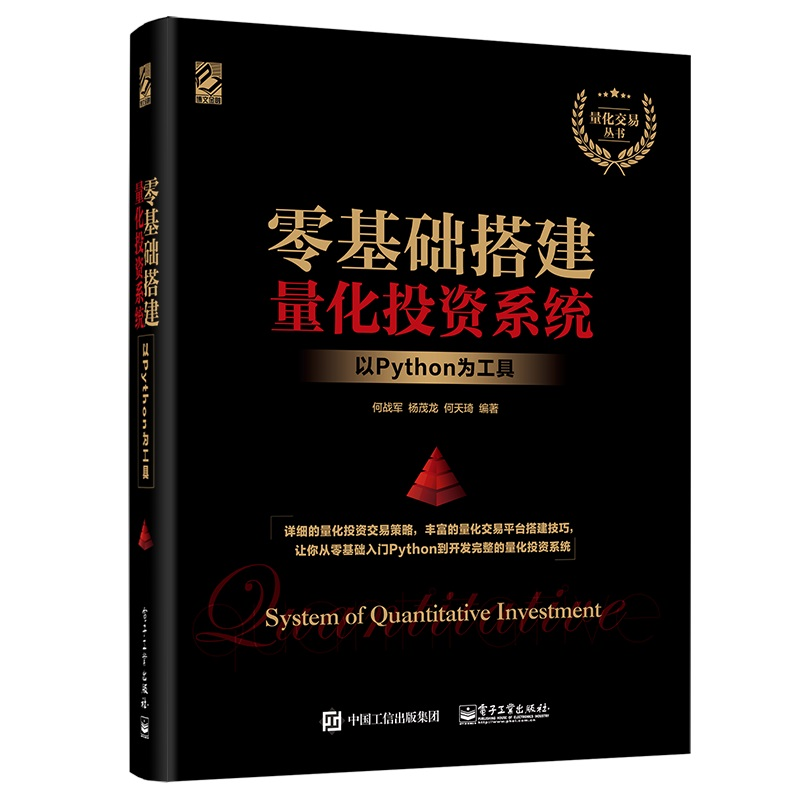书中提供的演示源码如下。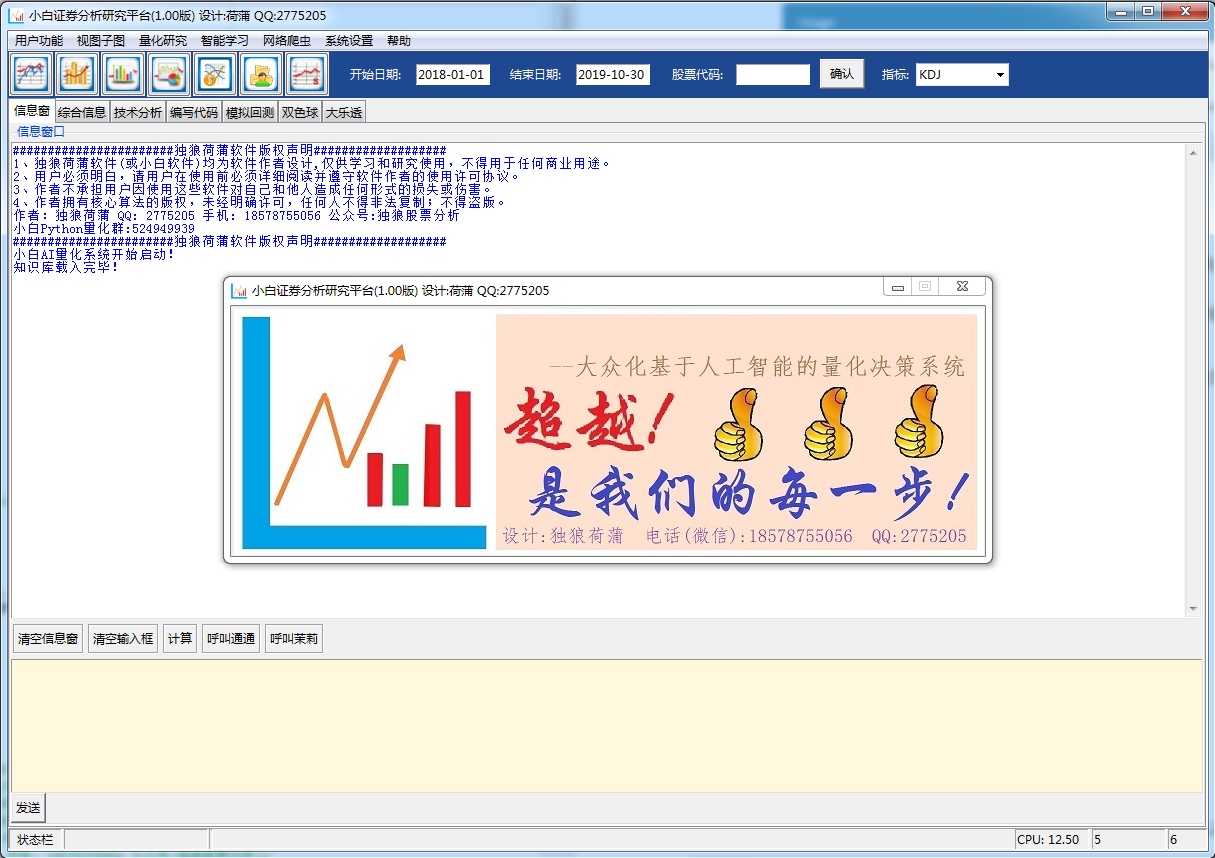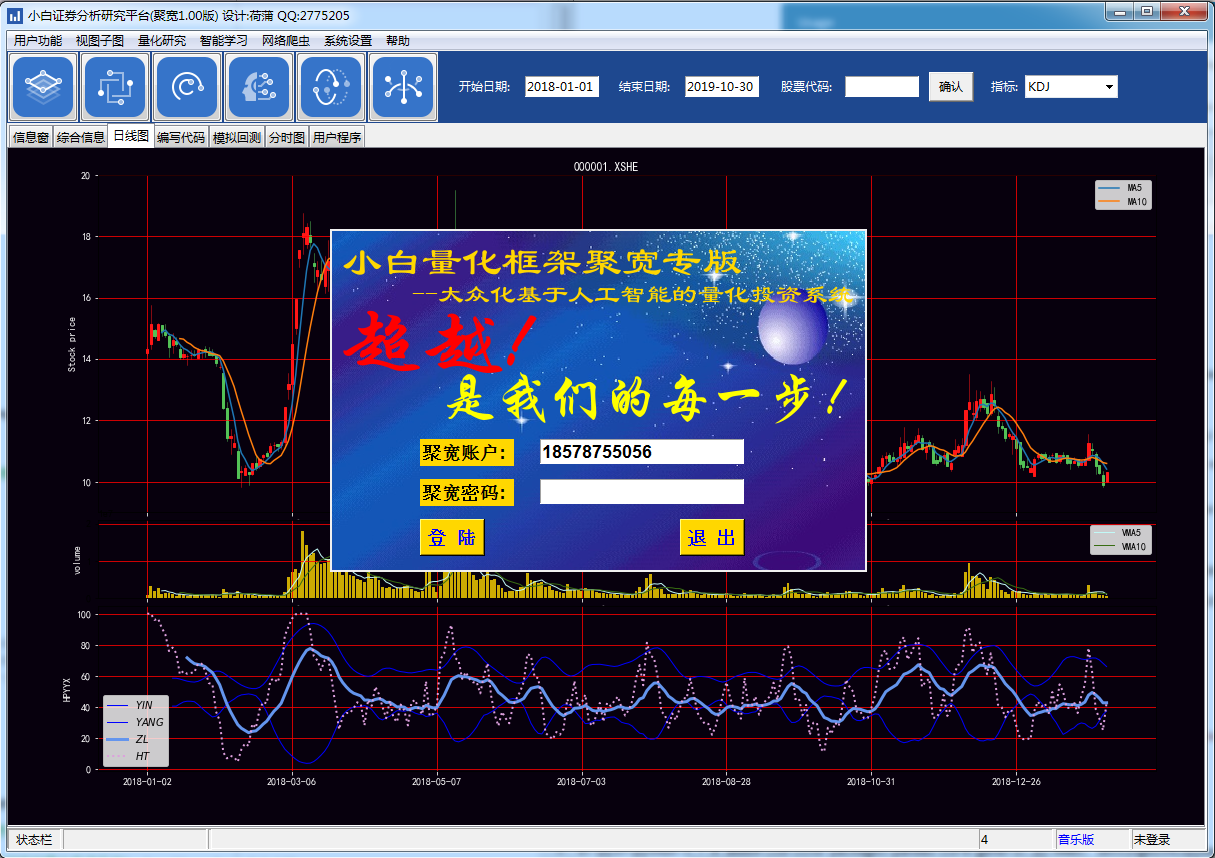上面2套源代码随书下载。支持多种行情源。 作者又为读者设计了新一代小白量化平台系统，支持pytdx实时行情、期货等行情。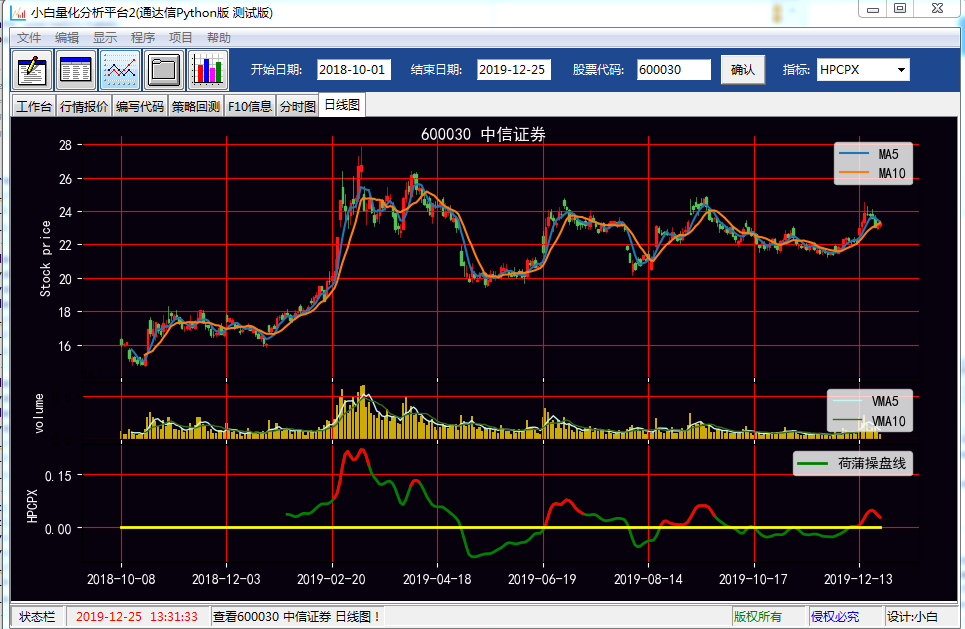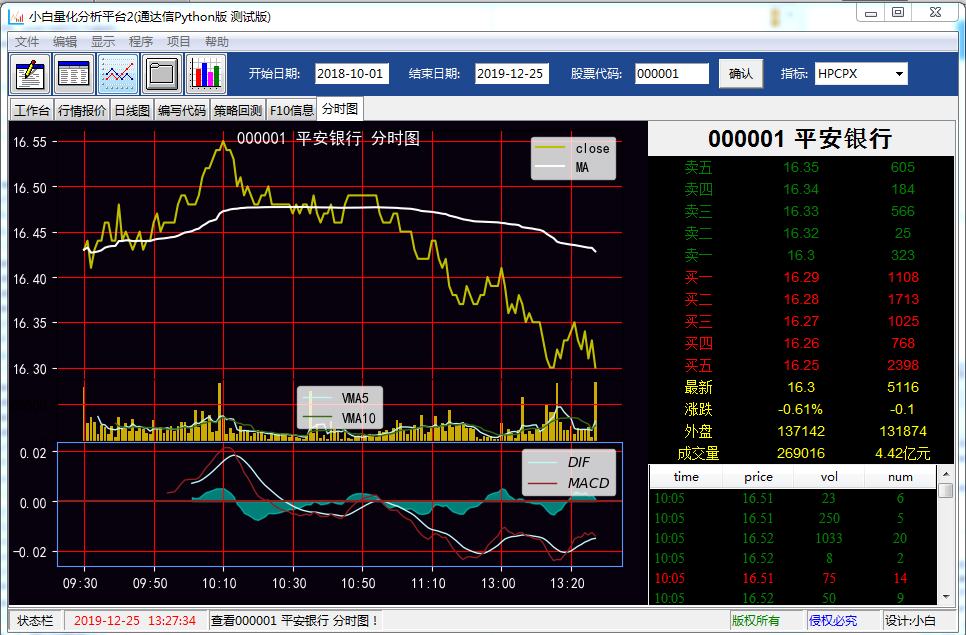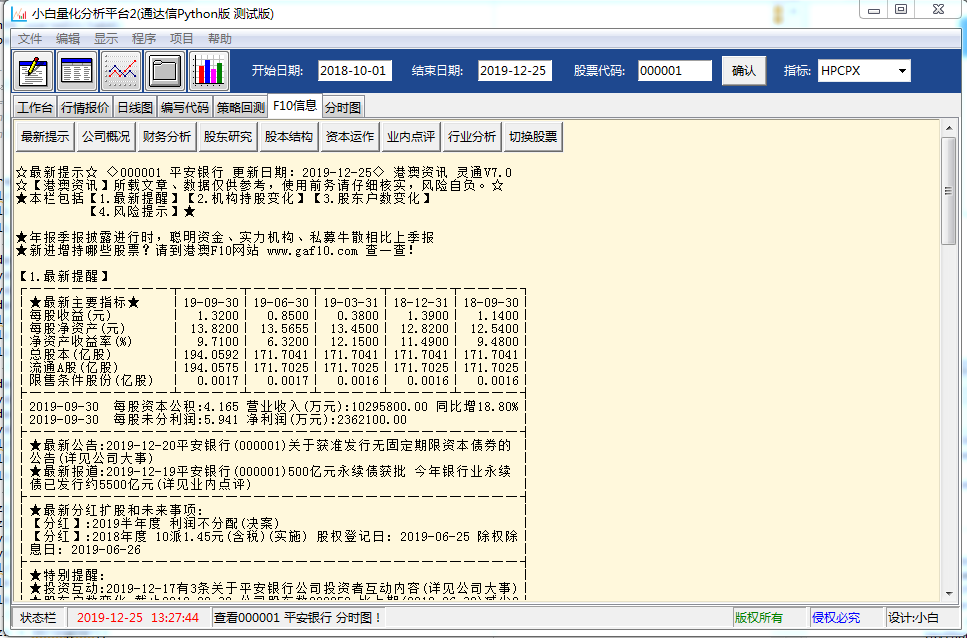#框架中[增强插件]在view目录中 #框架中[用户程序]在user目录中 #股票代码框输入:fst分时图 rxt日线图 f10 F10信息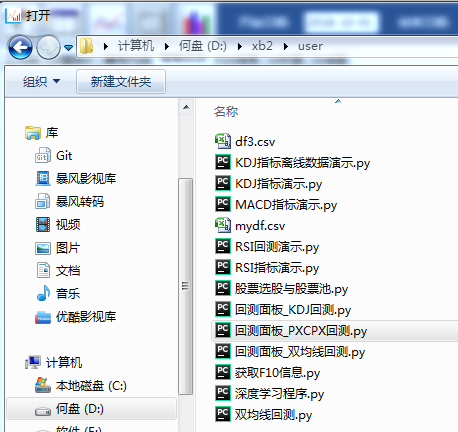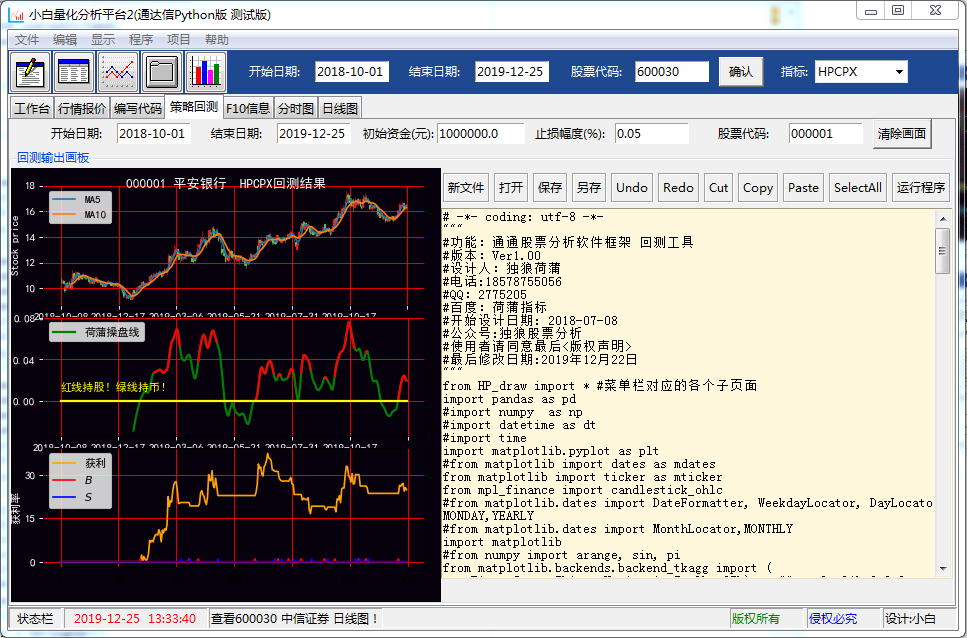下面我们看看[日线图]插件的写法。
# -*- coding: utf-8 -*-
#日线图.py
# 显示日线图的模板
import  tkinter  as  tk   #导入Tkinter
import  tkinter.ttk  as  ttk   #导入Tkinter.ttk
import  tkinter.tix  as  tix   #导入Tkinter.tix
import time
import pandas as pd
import numpy as np
import HP_global as g
import HP_data as hp
from HP_view import * #菜单栏对应的各个子页面
import HP_tdx as htdx

#系统设定了g.tab1--g.tab9,系统只是用了g.tab1--g.tab6
#控件结构 g.G_root -〉 g.tabControl  -〉g.tab1
#删除tab,用forget()
#当然用户可以设置更多的tab窗口。必须使用全局变量g.变量名
#重复建立新tab窗会出错，所以我们先检测是否None,不是就先做删除旧tab窗口。
g.gtype=g.book_s.get()
stockn=g.stock_i.get()
stockn=stockn.strip()
stockn=stockn.zfill(6)
stock.set(stockn)
g.stock=stockn
ds=date_s.get()
de=date_e.get()
g.sday=ds.strip()
g.eday=de.strip()
df2=htdx.get_k_data(g.stock,ktype='D',start=g.sday,\
end=g.eday,index=False,autype='qfq')
if g.tab3!=None:
g.tabControl.forget(g.tab3)
g.tab3=None

#用户自建新画面
g.tab3 = tk.Frame(g.tabControl)
g.tabControl.select(g.tab3)
axview3x(g.tab3,df2,t=g.stock+' '+g.stock_names[g.stock],n=2,f1='VOL',f2=g.gtype)
g.tabControl.select(g.tab3)
g.tabs=3

下面是KDJ回测代码。
# -*- coding: utf-8 -*-
"""
#功能：小白股票分析软件框架 回测工具
#版本：Ver1.00
#设计人：独狼荷蒲
#电话:18578755056
#QQ：2775205
#百度：荷蒲指标
#开始设计日期: 2018-07-08
#公众号:独狼股票分析
#使用者请同意最后<版权声明>
#最后修改日期:2019年12月22日
"""

global CLOSE,LOW,HIGH,OPEN,VOL
ds=g.hcdate_s.get()  #获取框架开始日期
de=g.hcdate_e.get()  #获取框架结束日期
stockn=g.hcstock.get()  #获取框架股票代码
df2=htdx.get_k_data(stockn,ktype='D',start=ds,end=de,index=False,autype='qfq')
df3=df2

##数据规格化
df3.dropna(inplace=True)

mydf=df3.copy()
CLOSE=mydf['close']
LOW=mydf['low']
HIGH=mydf['high']
OPEN=mydf['open']
VOL=mydf['volume']
C=mydf['close']
L=mydf['low']
H=mydf['high']
O=mydf['open']
V=mydf['volume']

##KDJ交易策略
def KDJ(N=9, M1=3, M2=3):
RSV = (CLOSE - LLV(LOW, N)) / (HHV(HIGH, N) - LLV(LOW, N)) * 100
K = SMA(RSV,M1,1)
D = SMA(K,M2,1)
J = 3*K-2*D
return K, D, J

k,d,j=KDJ(22,11,22)
mydf = mydf.join(pd.Series( k,name='K'))
mydf = mydf.join(pd.Series( d,name='D'))
mydf = mydf.join(pd.Series( j,name='J'))
mydf['S80']=80  #增加上轨80轨迹线
mydf['X20']=20  #增加下轨20轨迹线
mydf['Z50']=50  #增加中轨50轨迹线

##下面开始生成RSI指标买卖点
##买点J上穿数值20
b1=CROSS(mydf['J'],mydf['X20'])
mydf = mydf.join(pd.Series( b1,name='B1'))

##卖点J下穿80
s1=CROSS(mydf['S80'],mydf['J'])

#卖点J下穿50
s2=CROSS(mydf['Z50'],mydf['J'])

#合并所有卖点信号
s3=s1 | s2

mydf = mydf.join(pd.Series( s1,name='S1'))
mydf = mydf.join(pd.Series( s2,name='S2'))
mydf = mydf.join(pd.Series( s3,name='S3'))

g.tabControl.select(g.tab5)
##回测
tt=hpQuant()   ##初始化类
tt.code=stockn  #证券代码，必须输入
tt.stop_loss_on=False    #关闭自动止损

print('\n打印持仓信息')
tt.PrintSecurity()   #打印持仓信息

if g.UserCanvas!=None:

g.UserPlot.cla()
g.UserPlot.close()
g.UserCanvas._tkcanvas.pack_forget()
g.UserCanvas=None

######下面是绘图
# 开启一个双图例的窗口，定义为211和212
fig=plt.figure(2, figsize=(12,8), dpi=80)
g.UserFig=fig
g.UserPlot=plt
ax1 = plt.subplot(211)
ax2 = plt.subplot(212)

# ax1（211窗口）
plt.sca(ax1)
#添加标题
ax_K(ax1,df3,stockn)
plt.suptitle(stockn+' '+g.stock_names[stockn]+'  KDJ回测结果')

# ax2（212窗口）
plt.sca(ax2)
df3.HL.plot(color='orange', grid=True,label="获利")
df3.B1.plot(color='red',label="$B$")
df3.S3.plot(color='blue',label="$S$")

plt.legend() # 显示图中右上角的提示信息。
ax2.grid(True)
ax2.axhline(0, color='blue')
plt.gca().yaxis.set_major_locator(mticker.MaxNLocator(prune='upper'))
ax2.xaxis.set_major_locator(mticker.MaxNLocator(8))  #x轴分成几等分
ax2.yaxis.set_major_locator(mticker.MaxNLocator(nbins=3, prune='upper'))
plt.close()
canvas =FigureCanvasTkAgg(fig, master=g.UserFrame)

canvas.get_tk_widget().pack(side=tk.TOP, fill=tk.BOTH, expand=1)
g.UserCanvas=canvas
canvas._tkcanvas.pack(side=tk.TOP, fill=tk.BOTH, expand=1)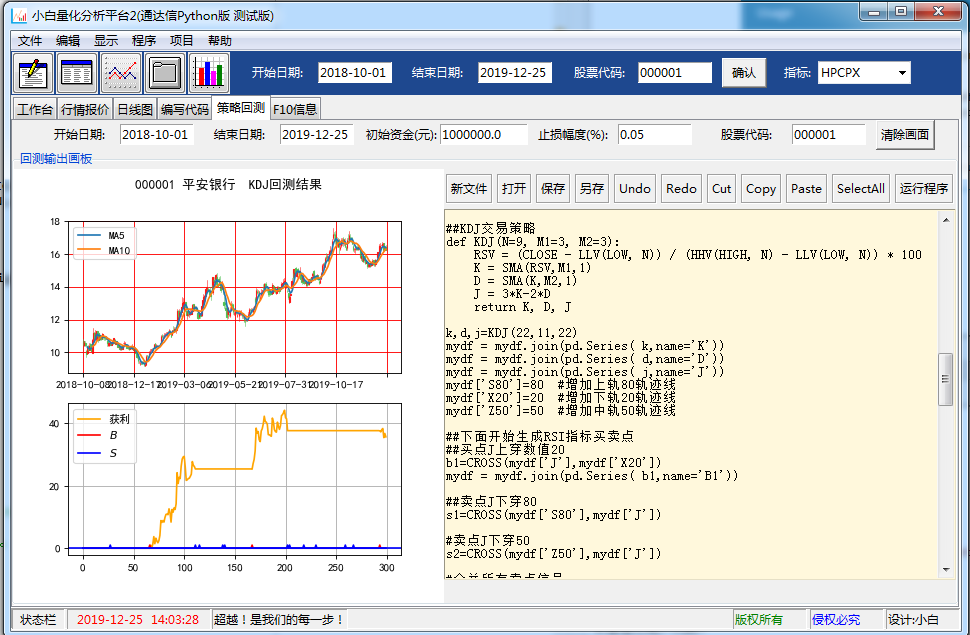独狼荷蒲qq:2775205 Python Tkinter小白量化群:524949939 电话微信:18578755056 微信公众号：独狼股票分析
其他QQ群： PythonTkinterGUI ： 517029284 Python学习交流： 647866213
展开全文python GUI
自己做量化交易软件(26)小白量化事件回测之MetaTrader5自动回测 我们前面文章介绍了小白量化的MetaTrader5行情分析和交易的知识，这篇文章，我们利用小白量化的事件回测模块来做一个MetaTrader5自动回测的演示。 我们利用了下列小白模块。 小白量化的事件回测模块HP_quant 小白量化MetaTrader5的模块HP_mt5 小白量化的公式函数库模块HP_formula 当然我们也可以使用talib库，例如下面代码：
mydf['opentunmo']=talib.CDLENGULFING(OPEN, HIGH, LOW, CLOSE)  #-100 为向下吞没, 100为向上吞没
upperband, middleband, lowerband=talib.BBANDS(CLOSE, timeperiod=20, nbdevup=2, nbdevdn=2, matype=0)
mydf['upperband']=upperband
mydf['middleband']=middleband
mydf['lowerband']=lowerband

我们下面给出的回测程序仍然使用小白量化的公式函数库模块HP_formula，这样方便用户使用自定义的公式指标。下面直接给出源代码，其中都作的注释。
# encoding:utf-8
'''
独狼荷蒲qq:2886002
通通小白python量化群:524949939
tkinter,pyqt,gui,Python交流2群:517029284
微信公众号：独狼股票分析
'''
import datetime
import matplotlib.pyplot as plt
import pandas as pd
from pandas.plotting import register_matplotlib_converters
register_matplotlib_converters()
import talib
import HP_mt5 as hmt5    #小白MT5模块
import HP_quant as hqu   #小白量化回测
from HP_formula import *   #小白公式函数模块

#买
data.price=data.close+context.dc
context.volume=volume
context.mode=1
context.price=data.price
context.s1=context.s1+1
print('买多')

#卖
def sell(context, data,volume=0.1):
data.price=data.close-context.dc
context.mode=2
context.volume=volume
context.price=data.price
context.s2=context.s2+1
print('买空')

#平仓
def pingcang(context, data):
if context.mode==1:
context.cash=context.cash+context.yingli
else:
context.cash=context.cash+context.yingli
context.mode=0
context.s3=context.s3+1
print('平仓')

#止损
def zhisun(context, data):
context.cash=context.cash+context.kuisun
context.mode=0
context.s4=context.s4+1
print('止损')

# BOLL 布林带指标
def BOLL(N=20, P=2):
"""
BOLL 布林带
"""
MID = MA(CLOSE, N)
UPPER = MID + STD(CLOSE, N) * P
LOWER = MID - STD(CLOSE, N) * P
return UPPER, MID, LOWER

G=hqu.GlobalVars()   #用户全局变量
context = hqu.Context()  #创建回测句柄
data = hqu.Data()  #创建交易数据

data.symbol='GOLD'   #回测品种
data.price=0.0  #成本价

def initialize(context,df):
print('回测初始化')
# 定义一个全局变量, 保存要操作的证券
context.stocks = ['GOLD']   #股票池
context.cash=2000.00    #现金
context.i=0        #i是起始位置
context.start=0        #i是起始位置
context.end=1500        #i是结束位置
df['day']=[ x[0:10] for x in df.date.astype(str)]
df['hour']=[ x[0:2] for x in df.time.astype(str)]
df['minute']=[ x[3:5] for x in df.time.astype(str)]
df['mode']=0  #买卖状态
df['yl']=0   #盈利
df['jz']=2000  #净值

df2=df[df.minute=='00']
df3=df2[df2.hour>='01']
df3=df3.reset_index(level=None, drop=True ,col_level=0, col_fill='')
day=df3.at[len(df3)-1,'day']
context.mydf=df    #df数据
context.day= day   #当前日期
context.yingli=5.0   #基准盈利
context.kuisun=-10.0  #基准亏损
context.volume=0.1   #持仓量
context.mode=0   #买单状态0:空仓,1:多单,2:空单
context.end=len(context.mydf)  #结束位置
context.start=0        #i是起始位置
context.dc=0.5   #点差
context.price=0.0   #成本价
context.s1=0   #买多次数
context.s2=0   #买空次数
context.s3=0   #平仓次数
context.s4=0   #止损次数

#------------------------------------------------------
pd.set_option('display.max_columns', 50) # number of columns to be displayed
pd.set_option('display.width', 1500)      # max table width to display
startTime=datetime.datetime.now()

print("initialize() failed")
mt5.shutdown()

if not mt5.initialize():
print("initialize() failed, error code =", mt5.last_error())
quit()

rates= mt5.copy_rates_from_pos("GOLD", mt5.TIMEFRAME_H1, 0, 10000)
data2=hmt5.tohpdata(rates)
data2['time']=[x[11:19] for x in data2.time.astype(str)]
print(data2)

mydf=data2.copy()
mydf=mydf.reset_index(level=None, drop=True ,col_level=0, col_fill='')
CLOSE= mydf['close']
HIGH=mydf['high']
LOW=mydf.low
OPEN=mydf.open

u,m,l=BOLL()
mydf['u']=u
mydf['m']=m
mydf['l']=l

mydf['ma3']=EMA(CLOSE,3)*0.995
mydf['ma3b']=EMA(CLOSE,3)*1.005
#买点策略,3日均线上穿布林指标下规
mydf['B']=CROSS(mydf['ma3'],mydf['l'])

#mydf['S']=CROSS(mydf['u'],mydf['ma3b'])
mydf['S']=0   #关闭卖出信号

#绘图
i=1

mydf2.u.plot.line(legend=True)
mydf2.m.plot.line(legend=True)
mydf2.l.plot.line(legend=True)
mydf2.ma3.plot.line(legend=True)
mydf2.ma3b.plot.line(legend=True)

#策略初始化
initialize(context,mydf)
context.end=len(mydf)
print(mydf)

#用户策略
def handle_data(context,data):
i=context.i

#获取每个数据的值
df3=context.mydf
day=df3.at[i,'day']
data.day=df3.at[i,'day']
data.high=df3.at[i,'high']
data.low=df3.at[i,'low']
data.open=df3.at[i,'open']
data.time=df3.at[i,'time']
data.close=df3.at[i,'close']
B=df3.at[i,'B']
S=df3.at[i,'S']

if context.mode==0 and B==1:  #买多
elif context.mode==0 and S==1:  #买空
sell(context, data)
elif context.mode==1:  #买多状态
if data.high-context.dc-data.price > context.yingli: #买多止盈
pingcang(context, data)
elif data.price-data.low-context.dc < context.kuisun:  #买多止损
zhisun(context, data)
elif context.mode==2:   #买空状态
if data.price-data.low+context.dc > context.yingli:  #买空止盈
pingcang(context, data)
elif data.high-data.price+context.dc < context.kuisun: #买空止损
zhisun(context, data)

context.i=i+1   #继续下一个周期

#开始回测
m=context.end-context.start

for i in range(context.start,context.end):
context.i=i
handle_data(context,data)
print('当前进度:',(i*100/m),data.day,data.time,'资金: ',context.cash)

print('买多:',context.s1,'买空:',context.s2,'止损:',context.s4,'资金:',context.cash)

#关闭MT5连接
mt5.shutdown()

下面看看运行结果：
当前进度: 99.87 2020-07-10 11:00:00 资金:  2420.0
当前进度: 99.88 2020-07-10 12:00:00 资金:  2420.0
买多
当前进度: 99.89 2020-07-10 13:00:00 资金:  2420.0
当前进度: 99.9 2020-07-10 14:00:00 资金:  2420.0
当前进度: 99.91 2020-07-10 15:00:00 资金:  2420.0
当前进度: 99.92 2020-07-10 16:00:00 资金:  2420.0
当前进度: 99.93 2020-07-10 17:00:00 资金:  2420.0
当前进度: 99.94 2020-07-10 18:00:00 资金:  2420.0
当前进度: 99.95 2020-07-10 19:00:00 资金:  2420.0
当前进度: 99.96 2020-07-10 20:00:00 资金:  2420.0
当前进度: 99.97 2020-07-10 21:00:00 资金:  2420.0
当前进度: 99.98 2020-07-10 22:00:00 资金:  2420.0
当前进度: 99.99 2020-07-10 23:00:00 资金:  2420.0
买多: 85 买空: 0 止损: 0 资金: 2420.0

显示出的一段图形如下：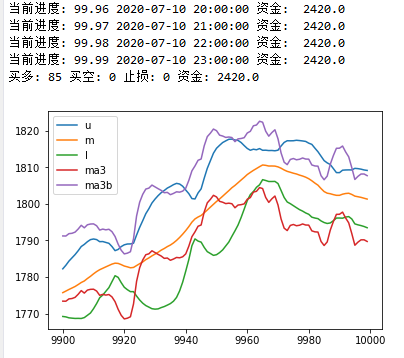以上文章介绍了MetaTrader5的Python方面的程序开发。MetaTrader5提供了外汇，期货，数字币等行情数据。读者不难结合前面的文章，根据自己策略写出全自动交易程序。 独狼荷蒲qq:2886002 通通小白python量化群:524949939 微信公众号：独狼股票分析
小白量化回测框架，是我们为<零基础搭建量化投资系统――以Python为工具>读者提供的源代码模块库，使用者可以任意改进和修改。一些读者利用小白量化模块，为自己搭建了特色回测程序，还有的读者利用这套小白量化模块为自己搭建了web股票数据网站，通过小白量化模块的仿通达信和仿大智慧，仿飞狐交易师的自编指标功能，以网站形式为用户展示了指标运算的结果数据，同时隐藏了自己的逻辑算法。欢迎大家在京东，淘宝等网站购买我们的正版书<零基础搭建量化投资系统――以Python为工具>，加入读者群，一起来完善小白量化平台，共同研究量化技术，一起提高和发展。
展开全文python
• 自己做量化交易软件(30)小白量化实战4–动于阴末止于阳极 　在讲本章之前，我先讲一个小故事。 　战国时，有个人乘船渡江，他上船时佩带的剑掉到了江里，他马上在船舷上刻了一个记号，然后从刻下记号的地方跳进水里...python gui
• 自己做量化交易软件(45小白量化实战18–直接使用通达信自编指标公式进行分析绘图和回测 小白量化一代提供了Python公式算法模式来写量化程序。 小白量化二代提供了仿通达信公式的模式来写量化程序。 小白量化三代除了...通达信公式 python 量化软件
• 自己做量化交易软件(35)小白量化实战8–事件型回测程序 小白量化第二代程序也提供了一个简单的事件型回测程序，我们前面的博客文章有介绍这方面的知识。当时用MT5 做的回测演示，见文章：自己做量化交易软件(26)小白...python
• 小白量化彩票实战（1）彩票分析及爬虫双色球彩票开奖数据 彩票选号码有两类：一是自主组号，二是软件算号。 1、自主组号。主要用自己认为的吉祥号,例如生日或有特殊意义,特殊感觉的号码。但是这些选出的号码多于彩票...python 爬虫
• 自己做量化交易软件(32)小白量化实战6–仿通达信公式选股 炒股也要有师傅。有一种低成本的师傅就是“书籍”了，这是市场中的投资名家经过多年实践总结出来的真知灼见，它可以使股民的投资水平陡然上升。事实上，很多...
• 自己做量化交易软件(44)小白量化实战17–利用小白量化金融模块在迅投QMT极速策略交易系统上仿大智慧指标回测及实战交易设计 小白量化平台是由若干小白金融模块构成。其中包含行情接收模块，仿通达信大智慧公式计算...指标公式 Python Tkinter
• 自己做量化交易软件(40)小白量化实战13–Alpha101及自编因子公式 2015年底World Quant发表了论文《101 Formulaic Alpha》。论文中给出了101个现实中的alpha。初期因子数据挖掘量化投资取得了不错的收益，后来实战...因子分析 Python Alpha101
• 自己做量化交易软件(25)小白量化用户Python代码编辑器 在量化软件设计中，经常会需要用户编写Python策略代码，因此需要在量化软件中有代码编辑器的功能。 我们想实现下图那样的功能，使用小白量化框架提供的模块很...python tkinter
• 自己做量化交易软件(24)小白量化增强仿通达信财务基本数据公式 通达信公式指标中有很多关于系统或基本面的函数，小白量化公式系统只有关于行情方面的公式基本函数。为了便于移植更多的自编通达信公式指标，我们给...python GUI
• 小白量化彩票实战（7）用sklearn神经网络预测彩票号码和特征 我写彩票的博客,不是鼓励大家去买彩票，读者要以学习编程和娱乐的思想来看待。兴趣是学习最大的动力！ 神经网络是目前比较热门的技术，人工神经网络...sklearn 神经网络 python
• 自己做量化交易软件(34)小白量化实战7–投资理念与通达信公式回测（2） 投资通俗地讲就是“财富变压器”。投资是使资本和财富生长的源泉。没有证券市场和期货市场时投资者当投出一元钱时，这一元钱资本压死在资产中...python GUIGUI 比特币python 自动交易
• 自己做量化交易软件(31)小白量化实战5–板块股票池与基本面选股 在小白量化软件中，股票池与板块是一样的,都是股票代码列表。前面博客有零散知识的介绍。小白量化学习(4)基本面选股与股票池 ...python java linux 算法 机器学习
• 小白量化学习（3）指标策略回测设计 一、准备工作 1、首先把如下文件复制到自己工程目录中。 小白量化Q群（小白量化群:524949939）共享中有下载。 HP_global.py HP_set.py HP_formula.py HP_sys.py 2、在新文件开始...Python
• 一、准备工作 1、首先把“HP_formula.py”文件复制到自己的工程目录中。...通过这2节学习，量化分析的道路已经为你开启了。 独狼荷蒲qq:2775205 通通python量化群:524949939 微信公众号：独狼股票分析Python GUI
• 小白量化入门步骤 金融知识 基本数理 —— 回归，概率，时间序列 编程 List item python -> 掌握Numpy和Pandas库，会一些算法最好。《利用Python进行数据分析》这本书就比较全了。 金融市场的数据分析，比较有...
• 小白量化学习（5）建立本地股票数据库 为了方便本地离线学习回测,可以在本地建立xbdata目录，存放股票数据。 一、本地建立xbdata目录 为了方便本地离线学习回测,可以在本地建立xbdata目录，存放股票数据。 ...Python GUI
• 自己做量化交易软件(39)小白量化实战12–利用神经网络程序预测价格上涨 大家使用小白量化主要目的不是为了什么仿通达信公式和仿大智慧公式,大家主要的目的想利用Python神经网络和深度学习等功能来用AI研究股市。从这...神经网络 python 机器学习 人工智能
• 小白量化学习（1）准备环境 一、为什么使用Python做量化平台。 （1）Python系统免费，有丰富的资源库，且跨不同操作系统平台。 （2）Python语法简单，且吸收了很多计算机语言的优点。 （3）Python是解释型交互式语言...Python GUI
• 这是《从编程小白量化宗师之路》系列的第一个高级课程。本课程宗旨是缩短个人和小型结构投资者和大型机构投资者的差距。课程内容从C++环境的安装开始使用，到期货数据采集，完美实现一套期货交易高频软件开发（也...C/C++ 期货高频
• 小白量化彩票实战（2）奖号特征值与编码器应用 我写彩票的博客,不是鼓励大家去买彩票，读者要以学习编程和娱乐的思想来看待。 索普玩的21点，只有1,2副牌，牌发完再重新洗牌，所以通过记牌，计牌估算概率，当形势...Python...